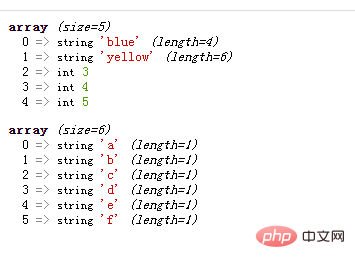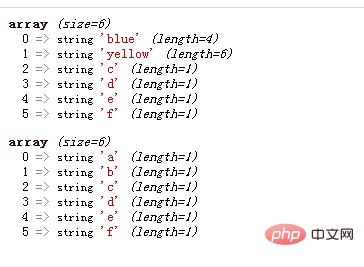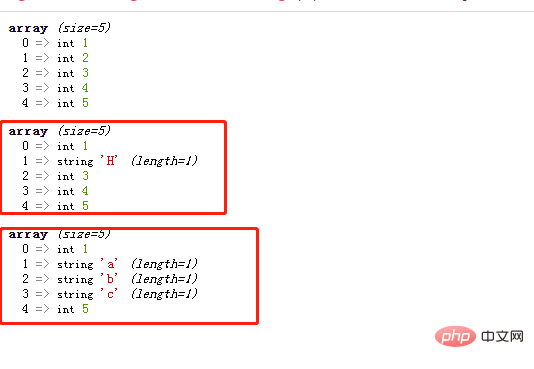# PHP怎么替换数组中元素PHP替换数组中元素的两种方法：

1、利用array_replace() 函数

array_replace() 函数使用后面数组的值替换第一个数组的值。

`array_replace(数组,替换数组)`

```<?php
\$a1=array(1,2,3,4,5);
\$a2=array("blue","yellow");
\$a3=array("a","b","c","d","e","f");
var_dump(array_replace(\$a1,\$a2));
var_dump(array_replace(\$a1,\$a3));
?>``````<?php
\$a1=array(1,2,3,4,5);
\$a2=array("blue","yellow");
\$a3=array("a","b","c","d","e","f");
var_dump(array_replace(\$a1,\$a3,\$a2));
var_dump(array_replace(\$a1,\$a2,\$a3));
?>```2、利用array_splice() 函数

array_splice()是一个强大的函数，可以实现删除、插入、替换元素操作。

`array_splice(\$array,\$start,\$length,\$replacement)`

• arr 表示一个数组。
• start 表示开始删除的位置（下标）：
• 如果 start 为正数，则从前往后删除。
• 如果 start 为负数，则从距离 arr 末端 -start 的位置开始，从后往前删除。例如 -2 意味着从数组的倒数第二个元素开始。
• length 是可选参数，表示删除的元素个数：
• 如果 length 为正数，那么就表示删除 length 个元素；
• 如果 length 为负数，那么将删除从 start 开始，到数组末尾倒数 length 为止的所有元素；
• 如果省略，那么将删除从 start 开始，一直到数组末尾的所有元素。
• replacement 是可选参数，表示要替换的值。如果 replacement 有多个值就需要设置为数组，如果只有一个值可以不设置为数组。

```<?php
\$arr=array(1,2,3,4,5);
var_dump(\$arr);
array_splice(\$arr,1,1,"H");
var_dump(\$arr);
array_splice(\$arr,1,3,array("a","b","c"));
var_dump(\$arr);
?>```1/1